# Selina Solutions Concise Mathematics Class 6 Chapter 16 Percent (Percentage)

Selina Solutions Concise Mathematics Class 6 Chapter 16 Percent (Percentage) helps students obtain a clear idea about the fundamental concepts explained here. Students are advised to solve the textbook problems on a regular basis to improve problem solving skills within a short duration. Solutions are designed by experts, with the aim to help them, in enhancing their academic performance. To build a stronghold on the concepts covered, students can use Selina Solutions Concise Mathematics Class 6 Chapter 16 Percent (Percentage) PDF, from the links provided below.

Chapter 16 provides students the method of solving the given statement as percent, along with suitable examples. Numerous examples before each exercise help students in solving the tricky problems effortlessly.

## Selina Solutions Concise Mathematics Class 6 Chapter 16: Percent (Percentage) Download PDF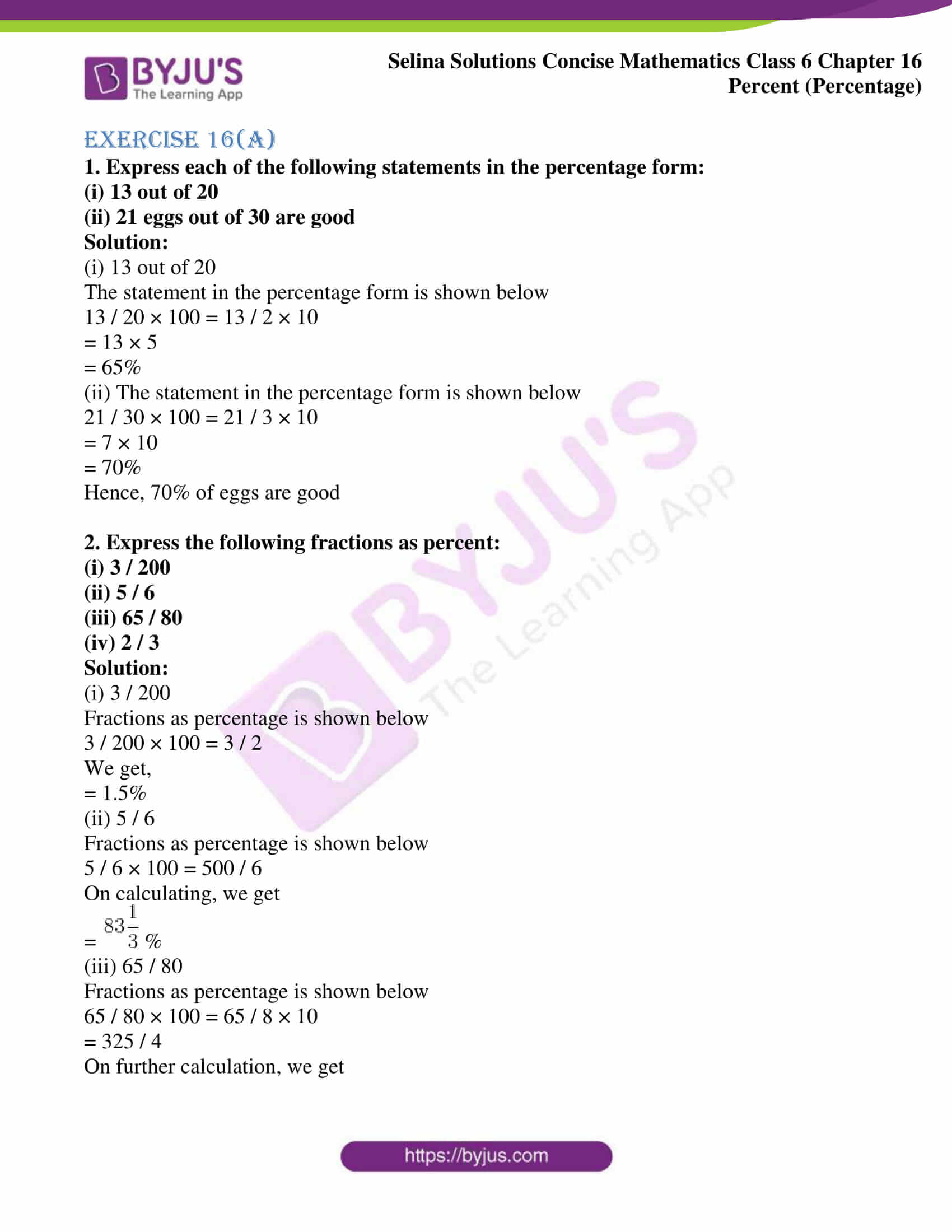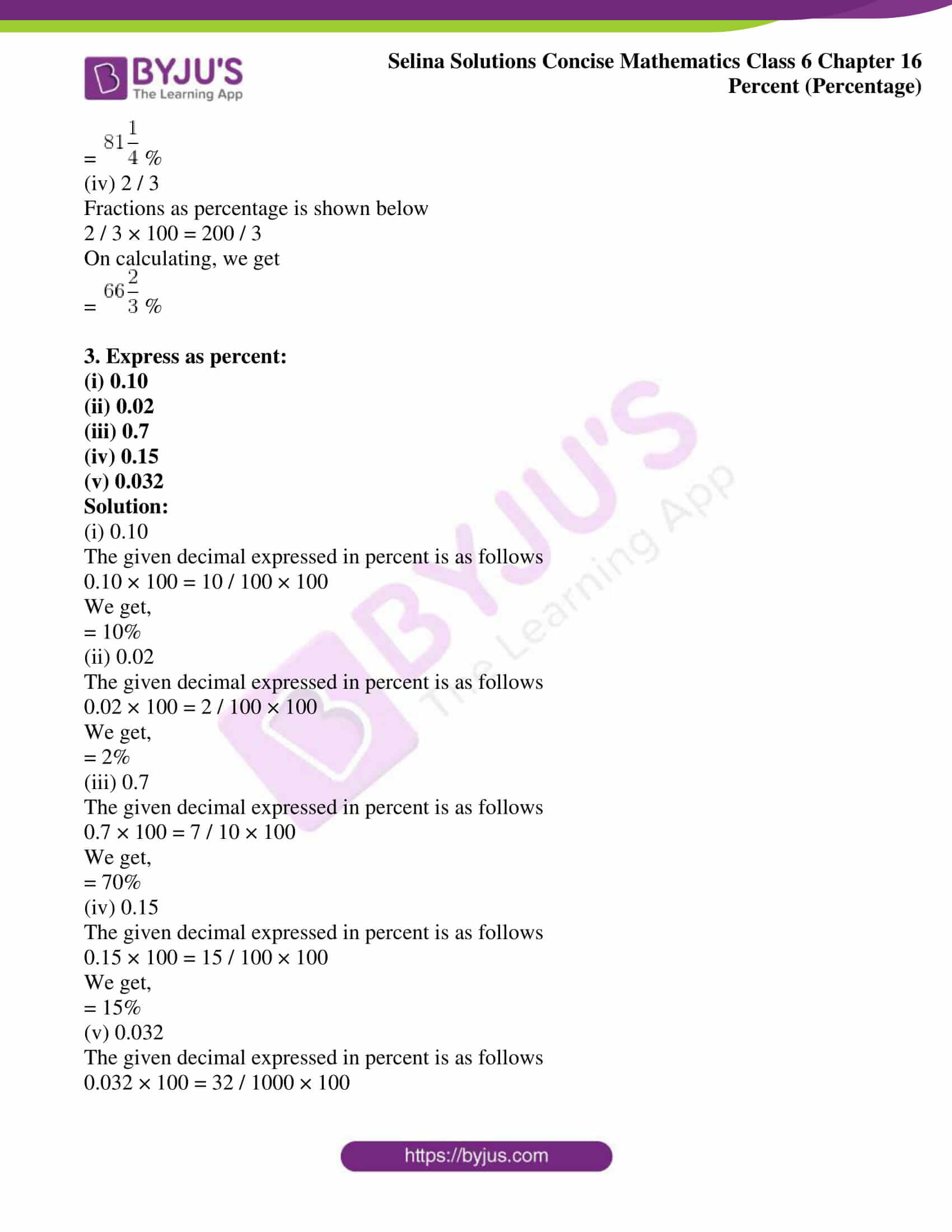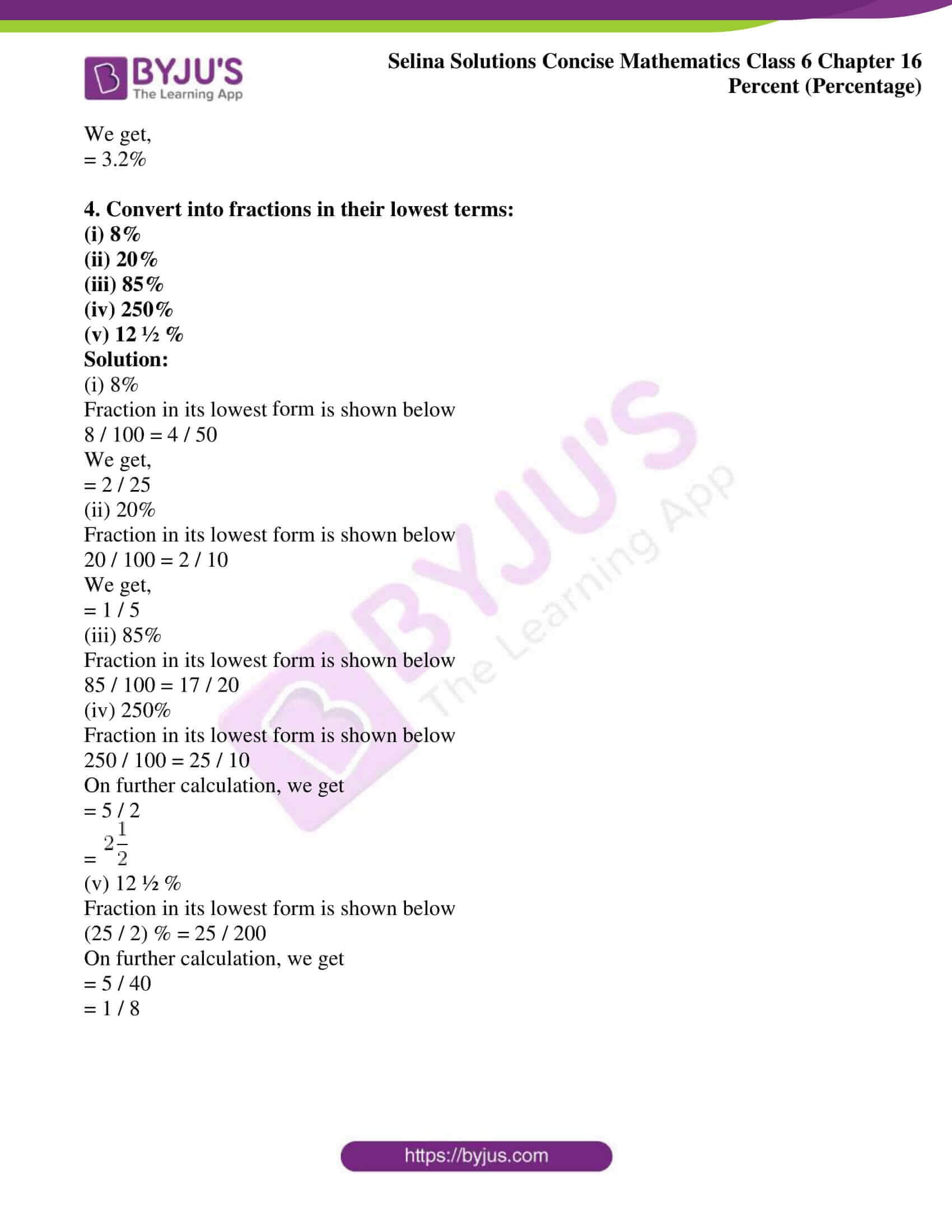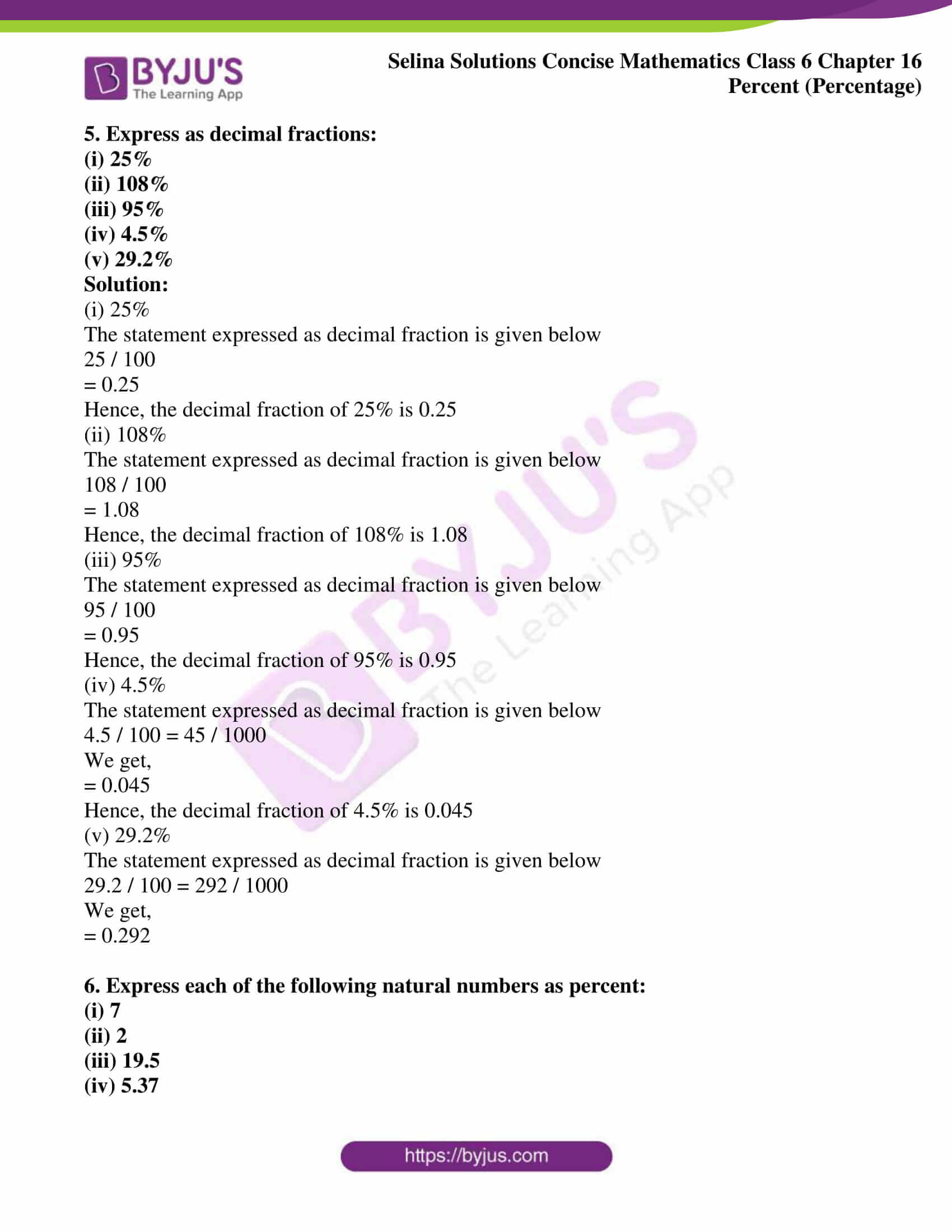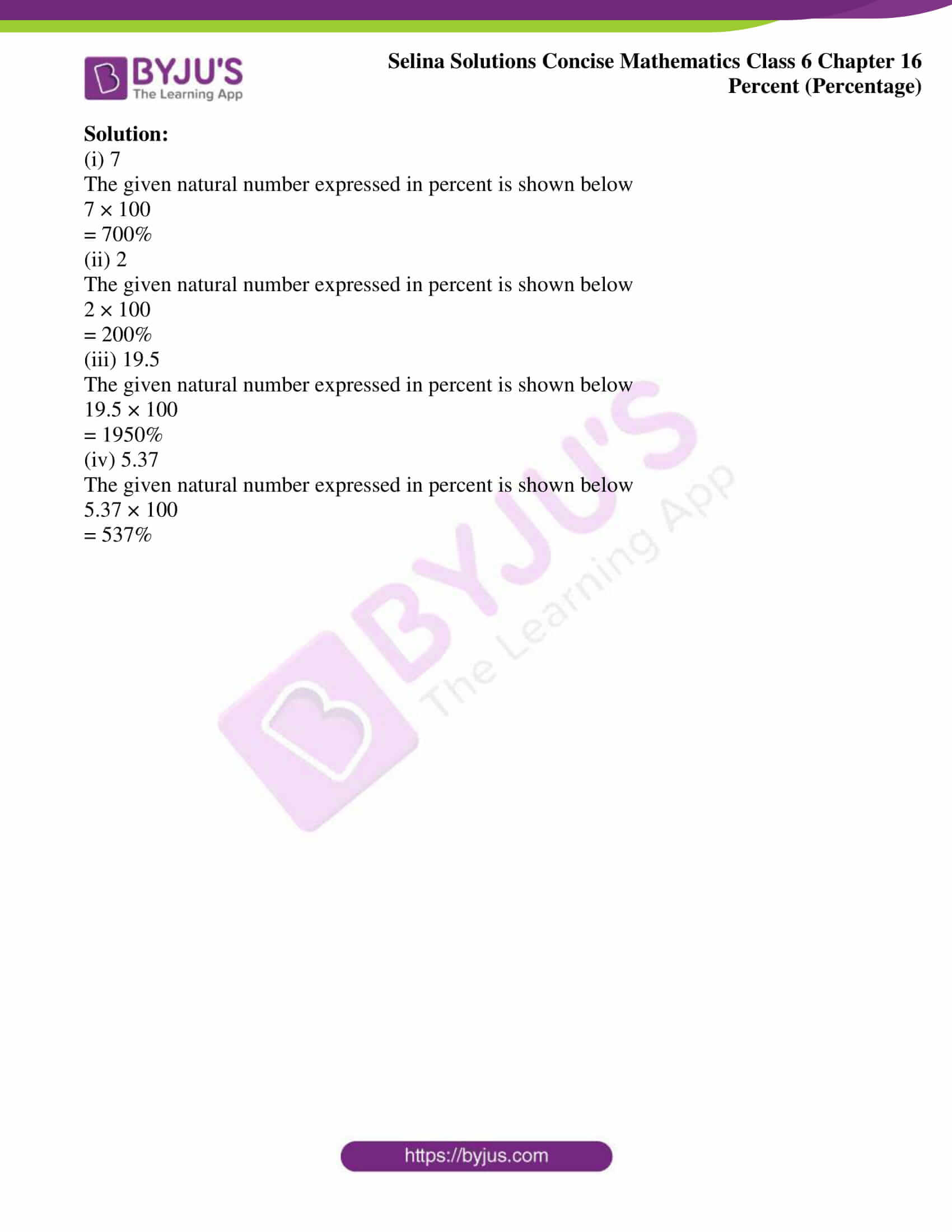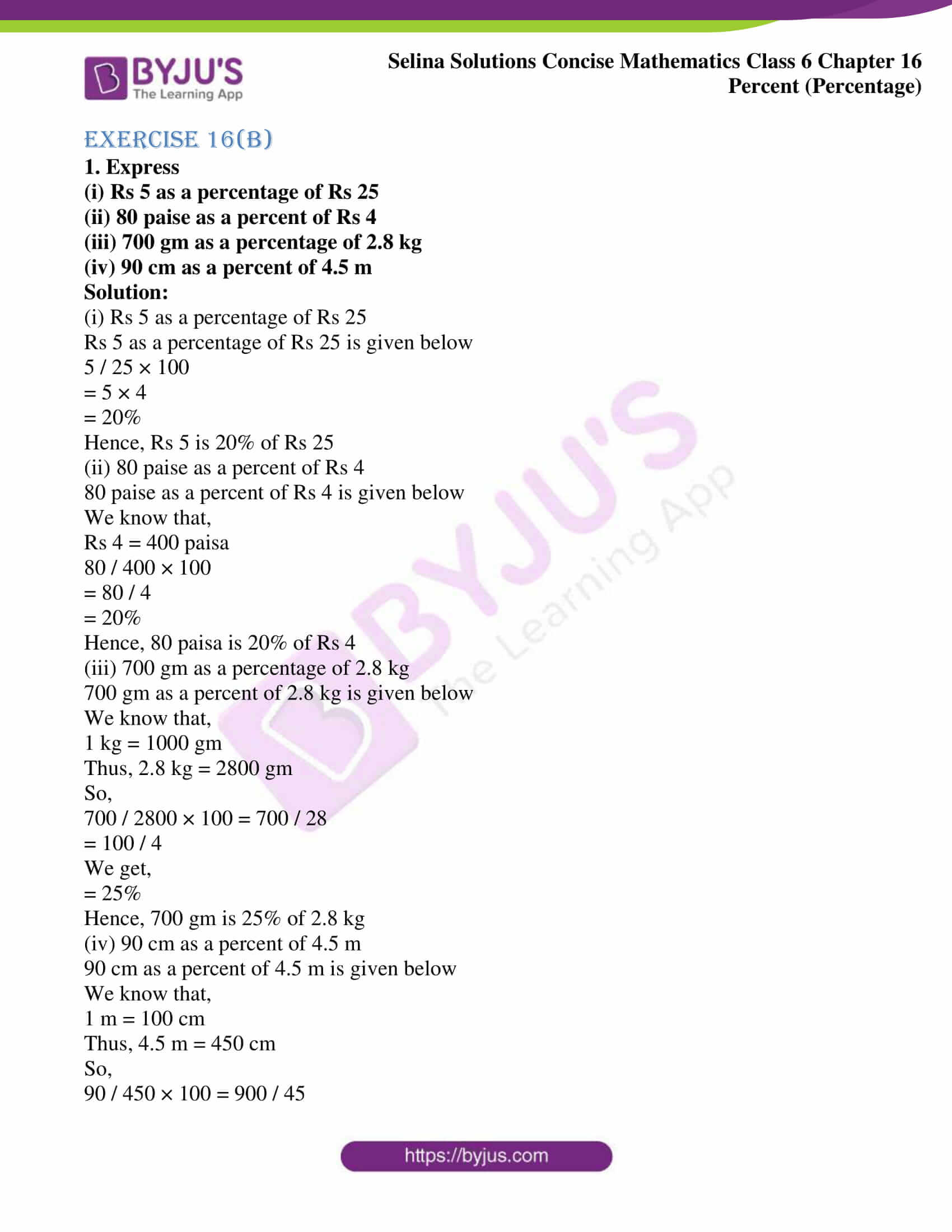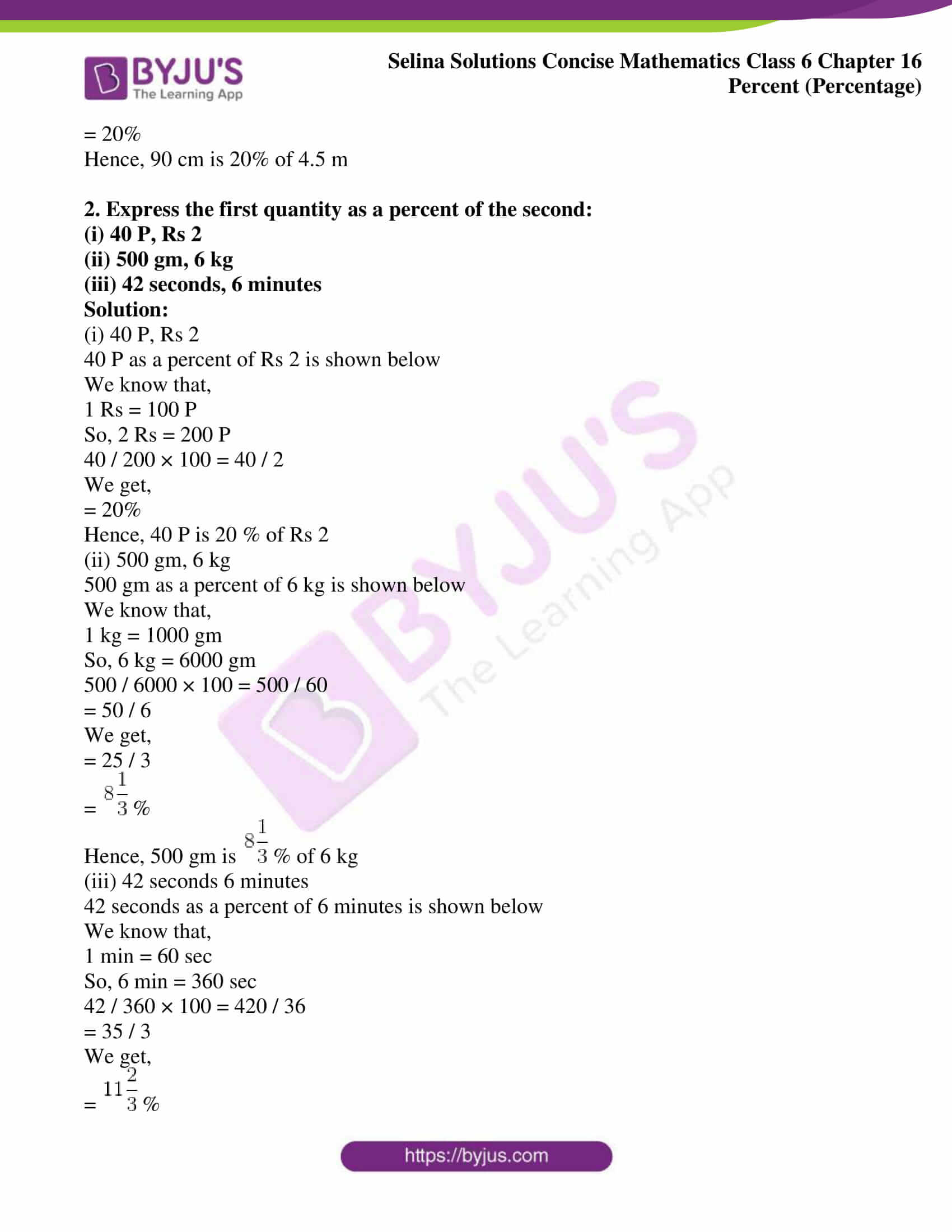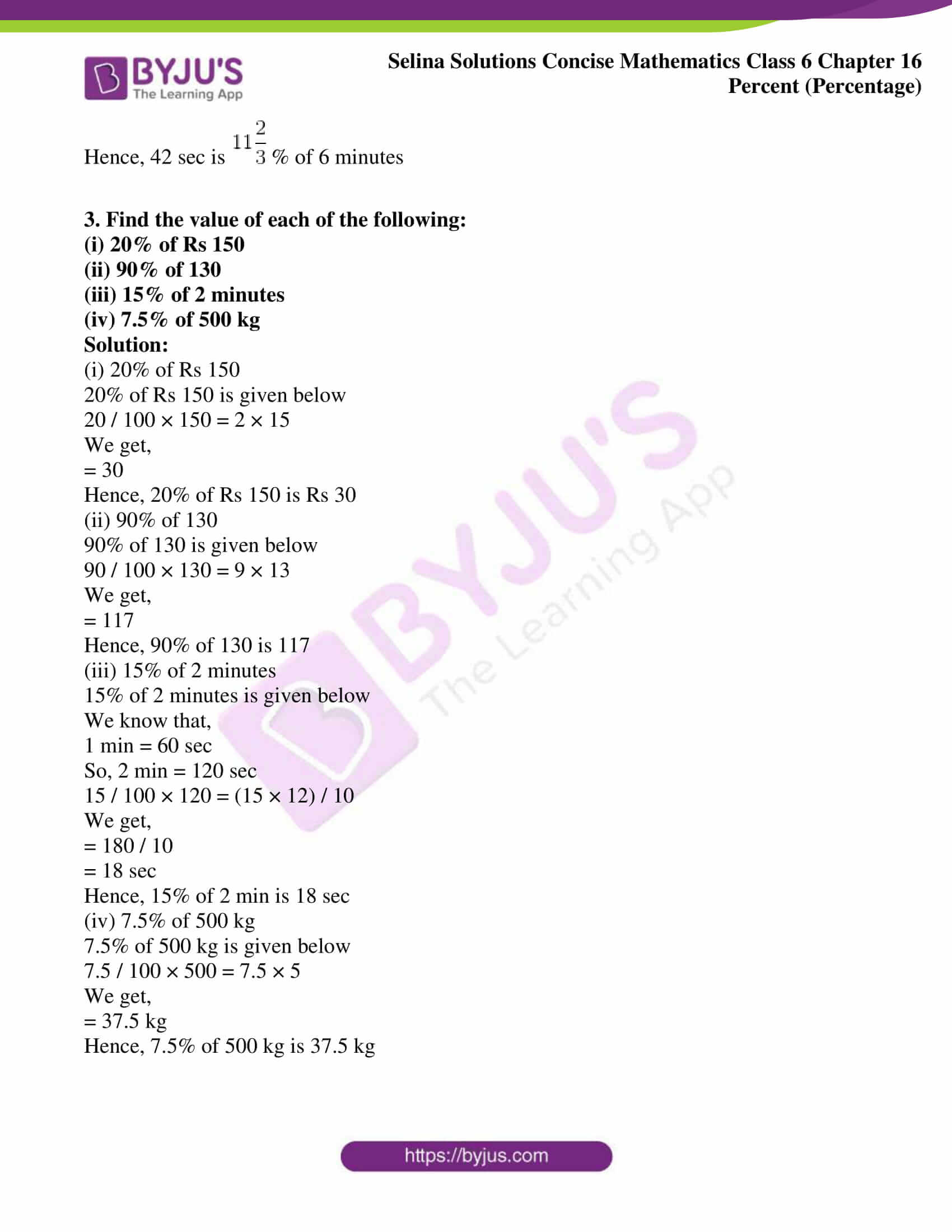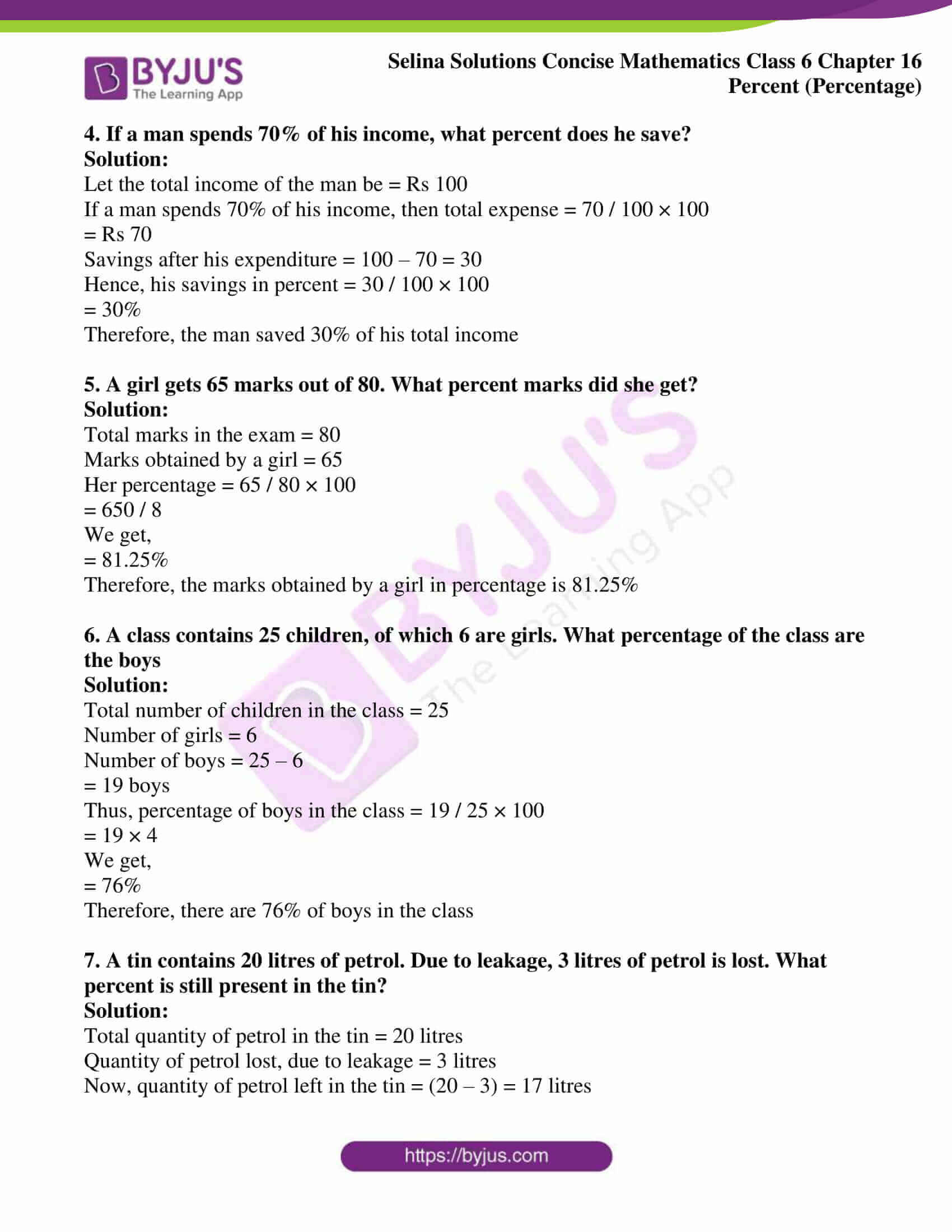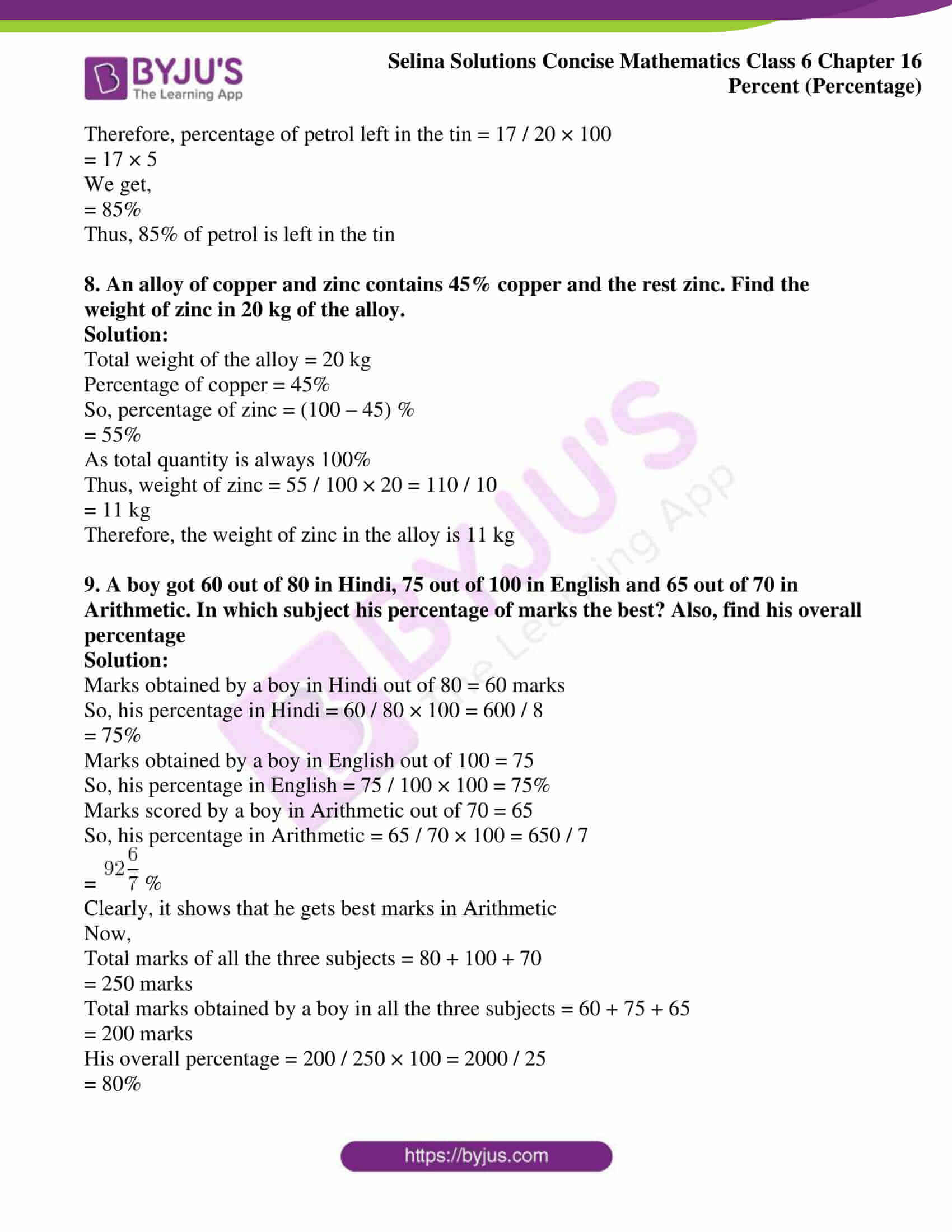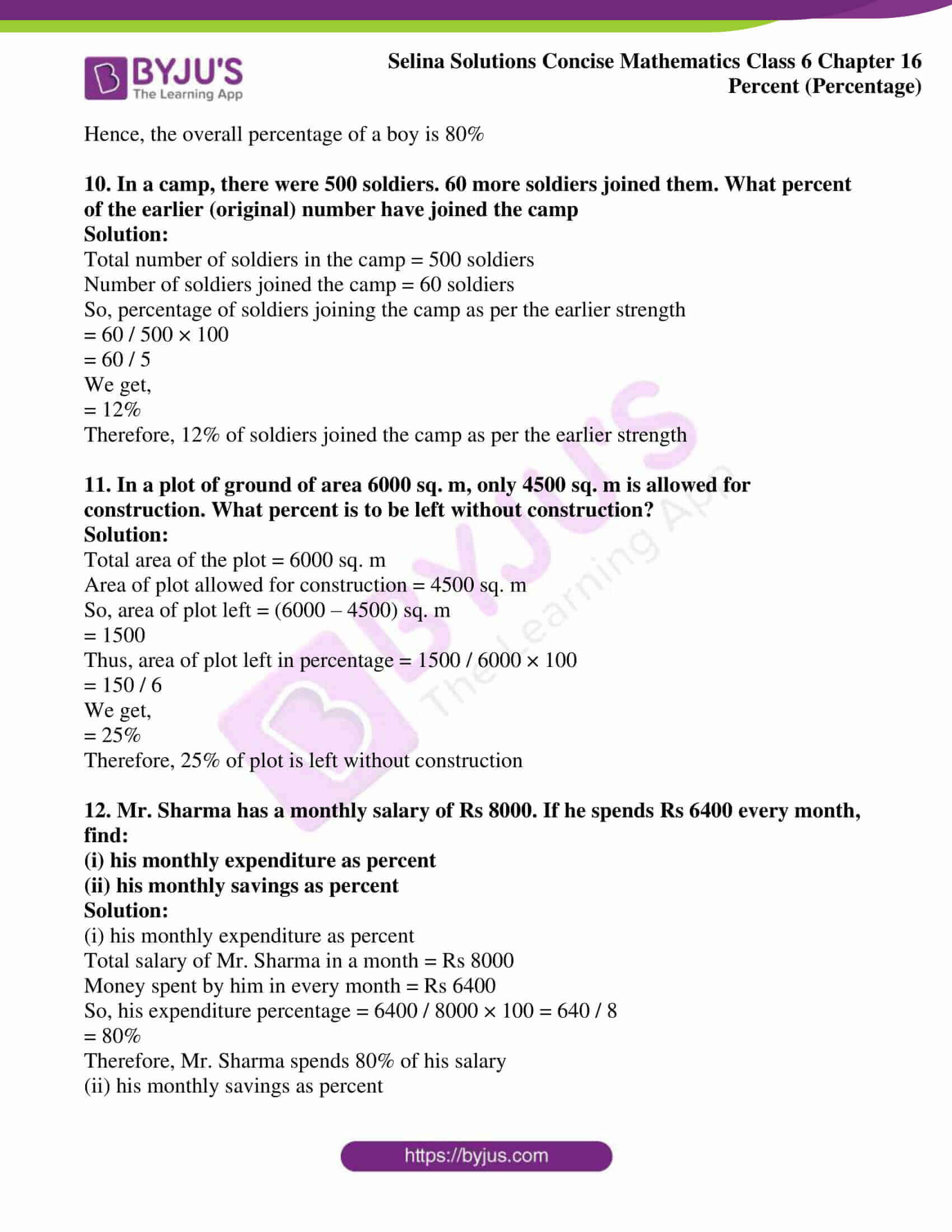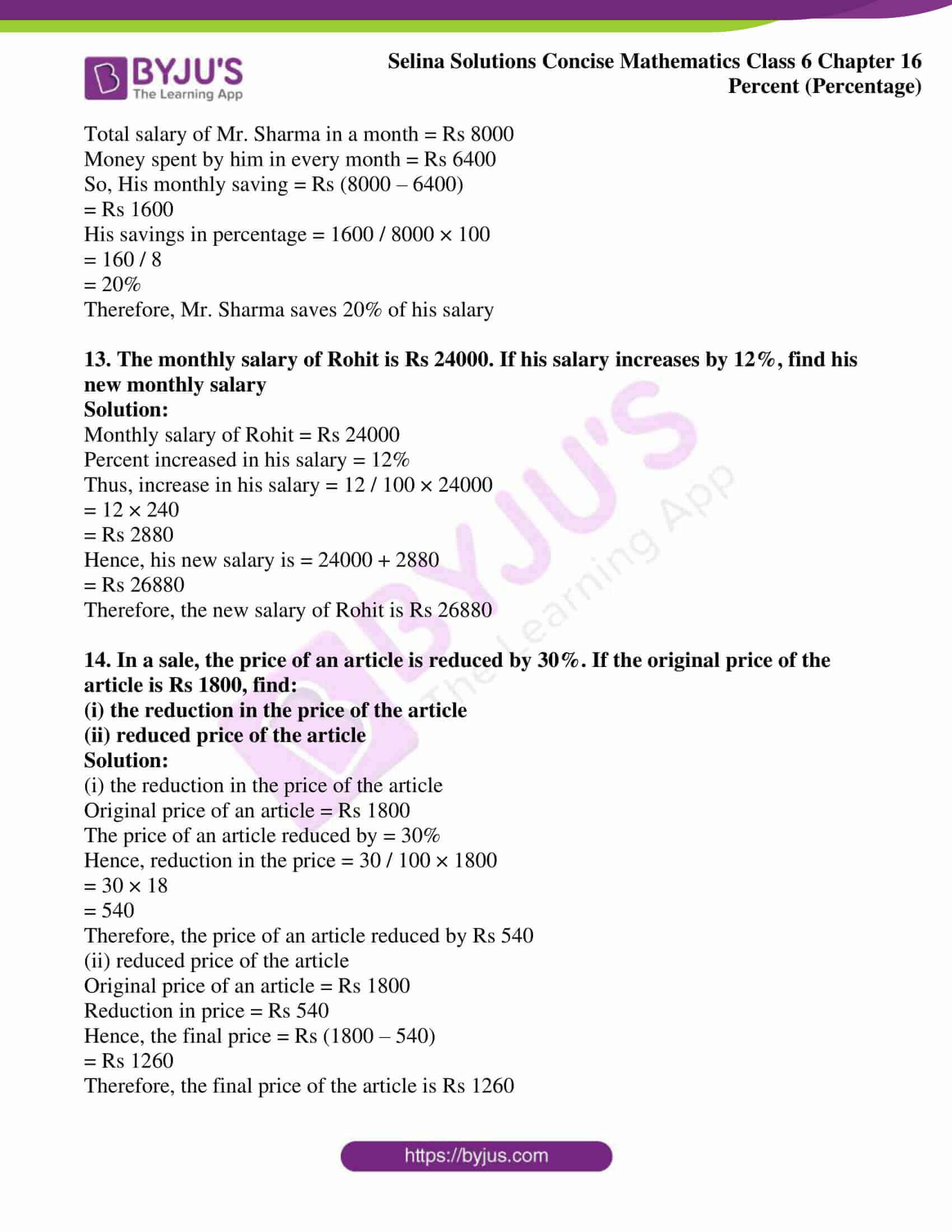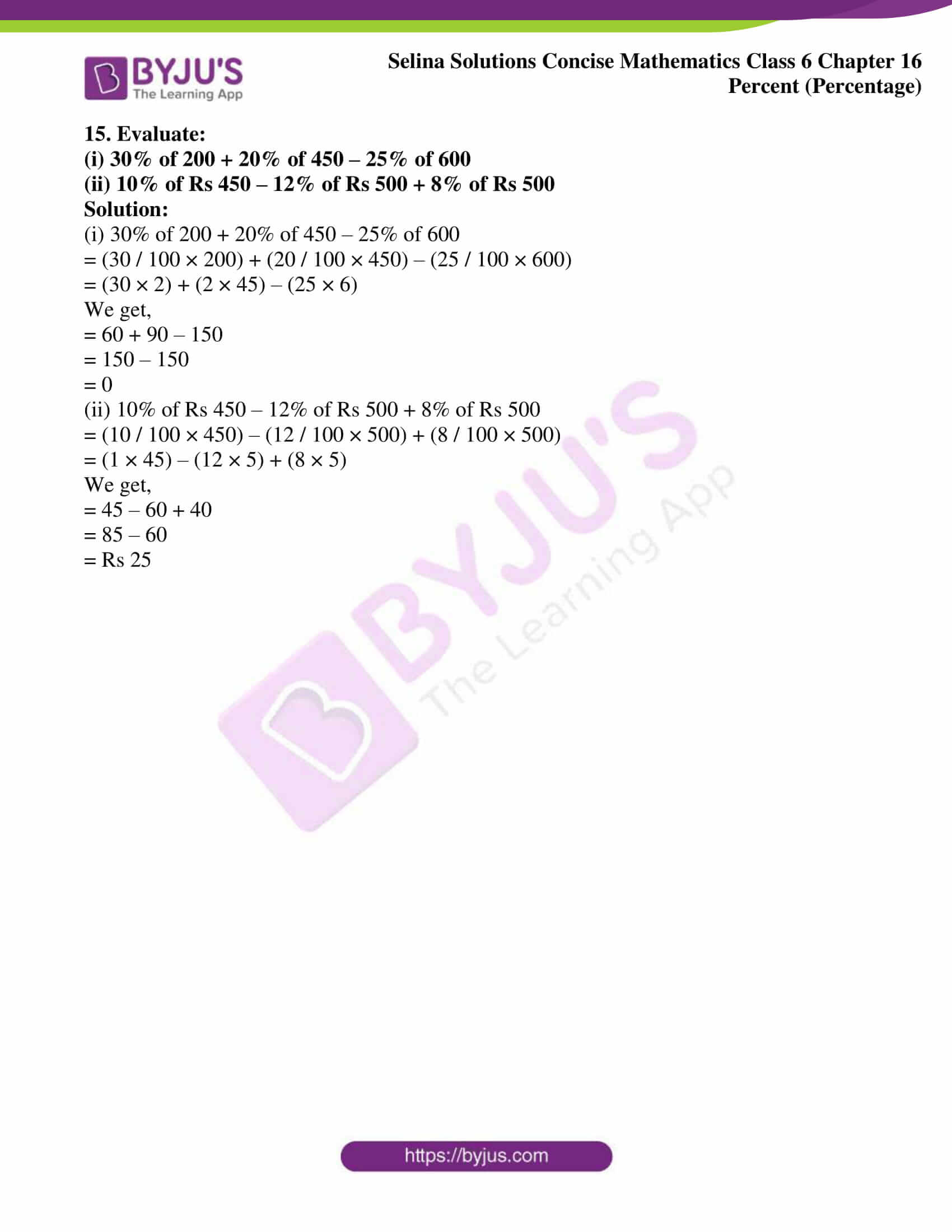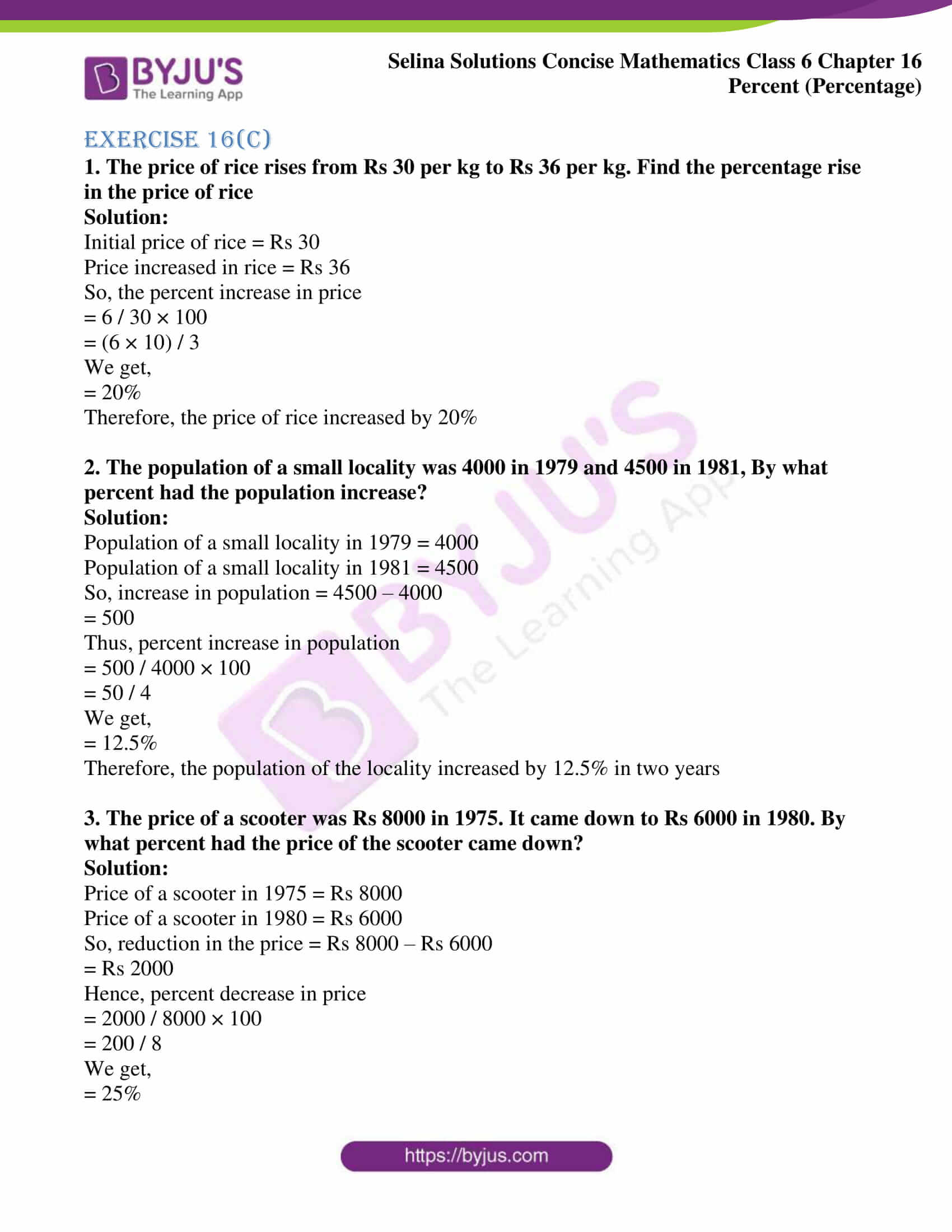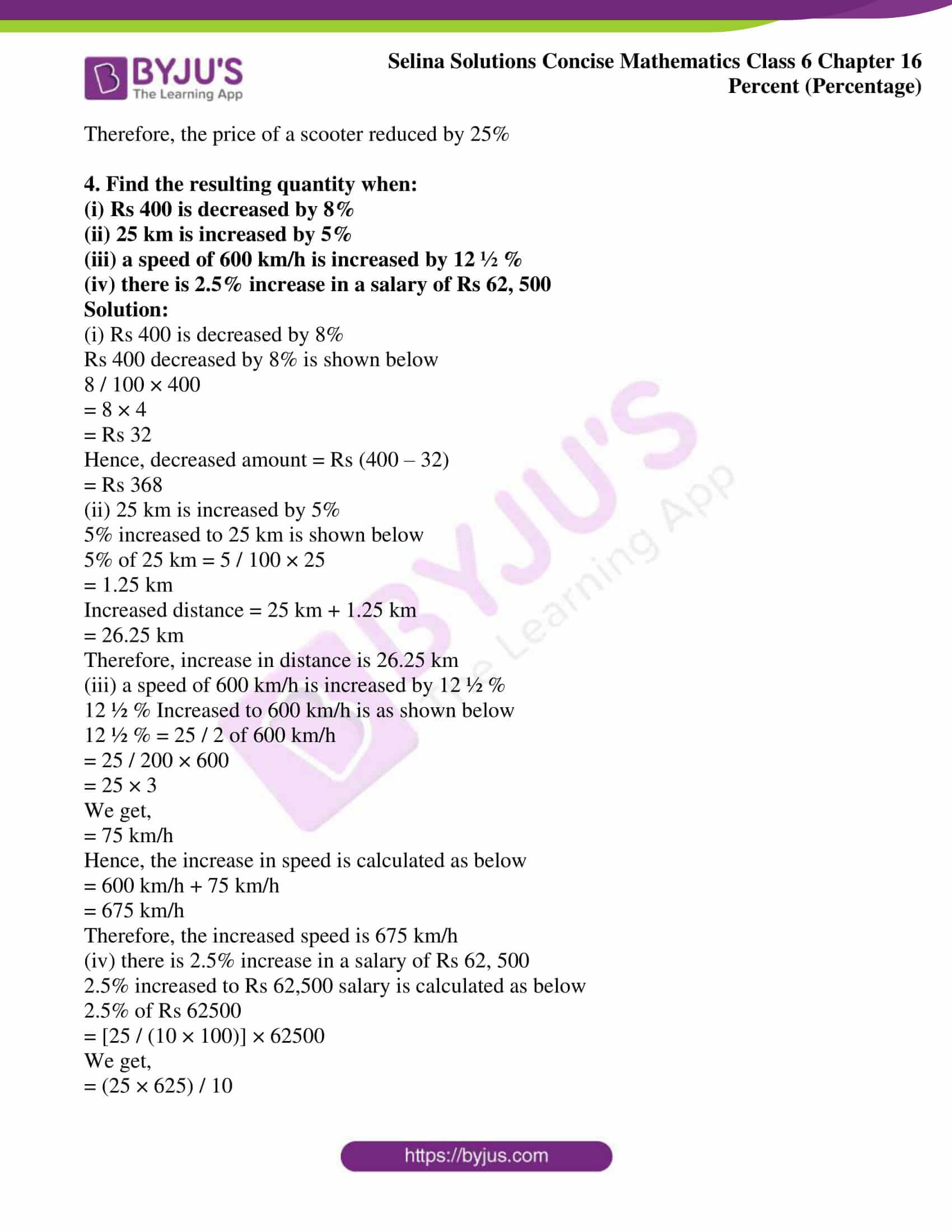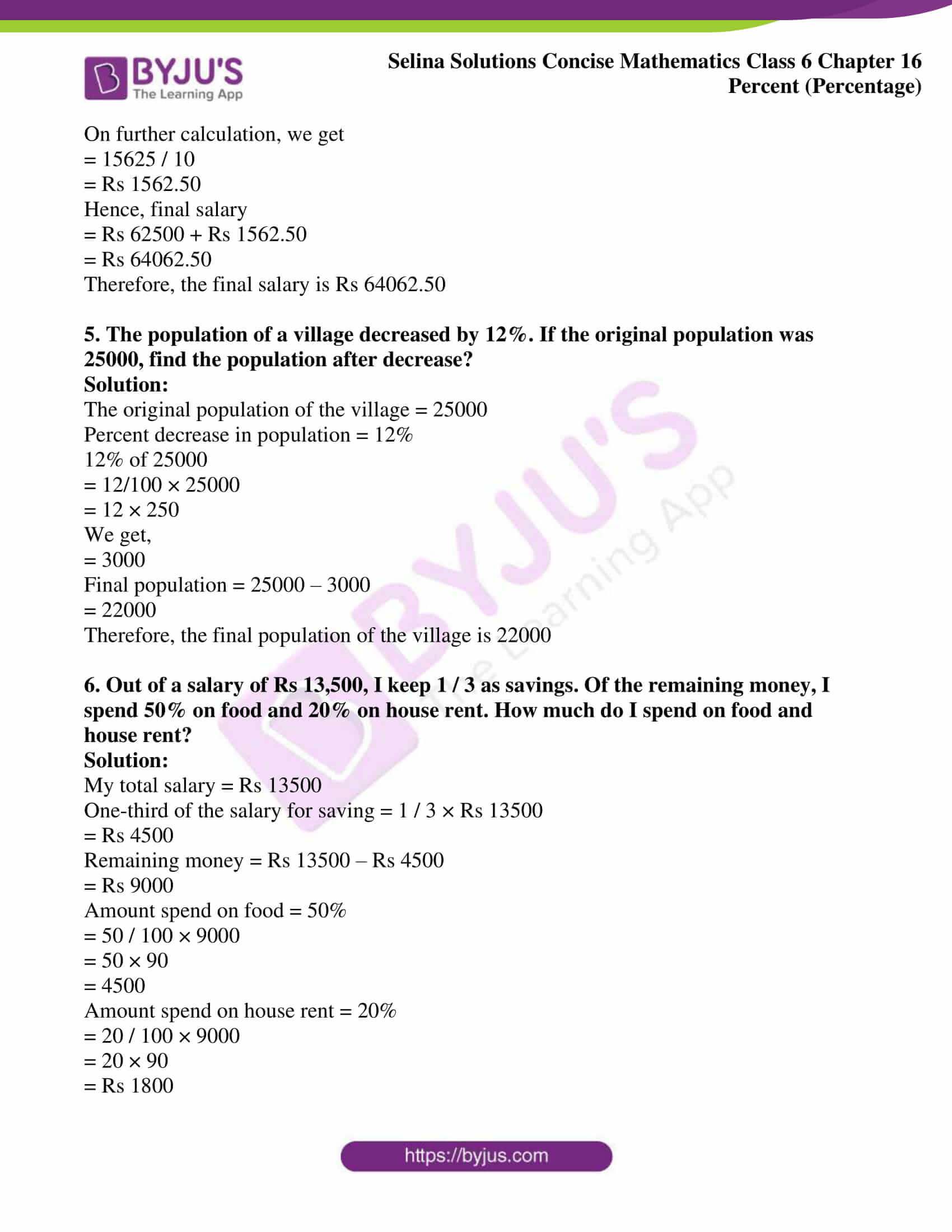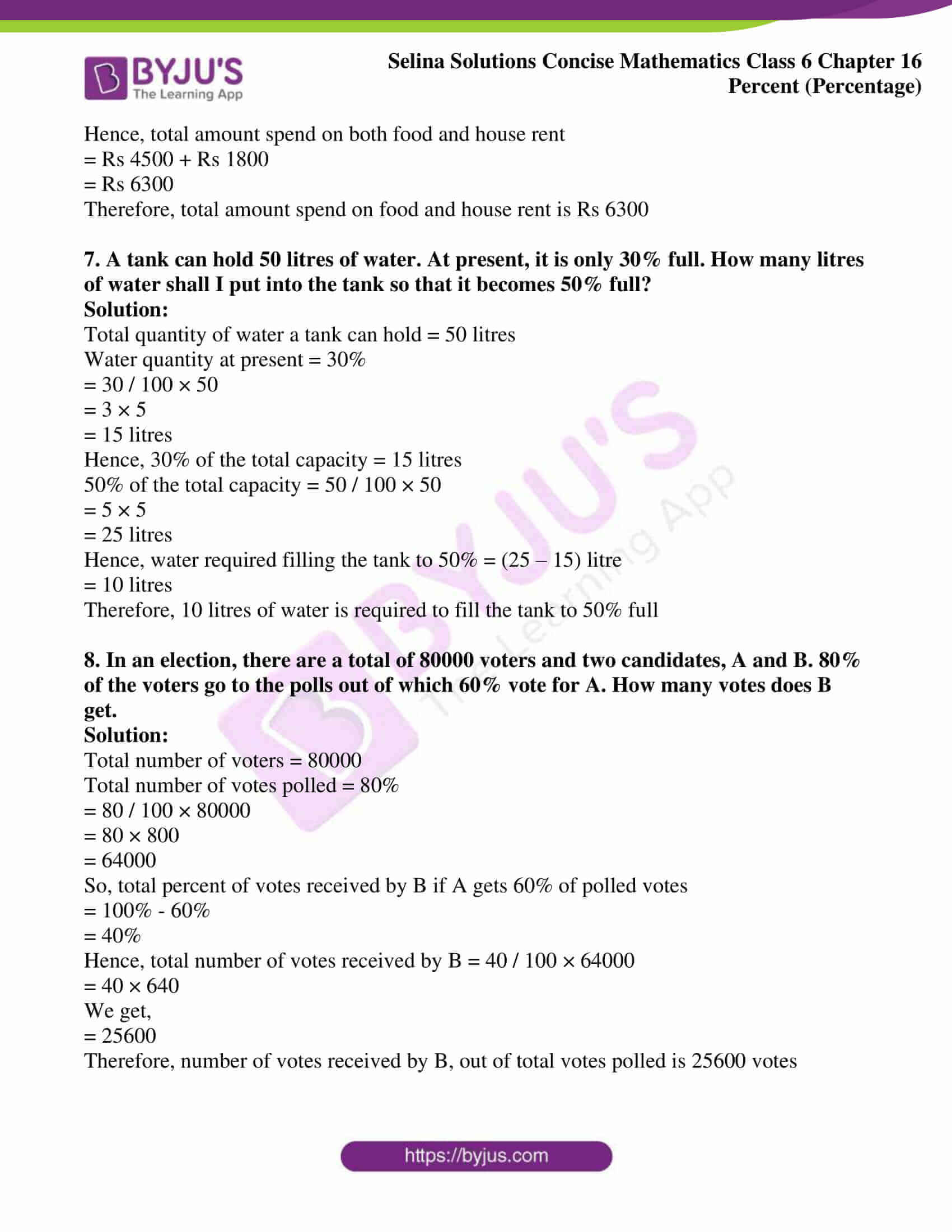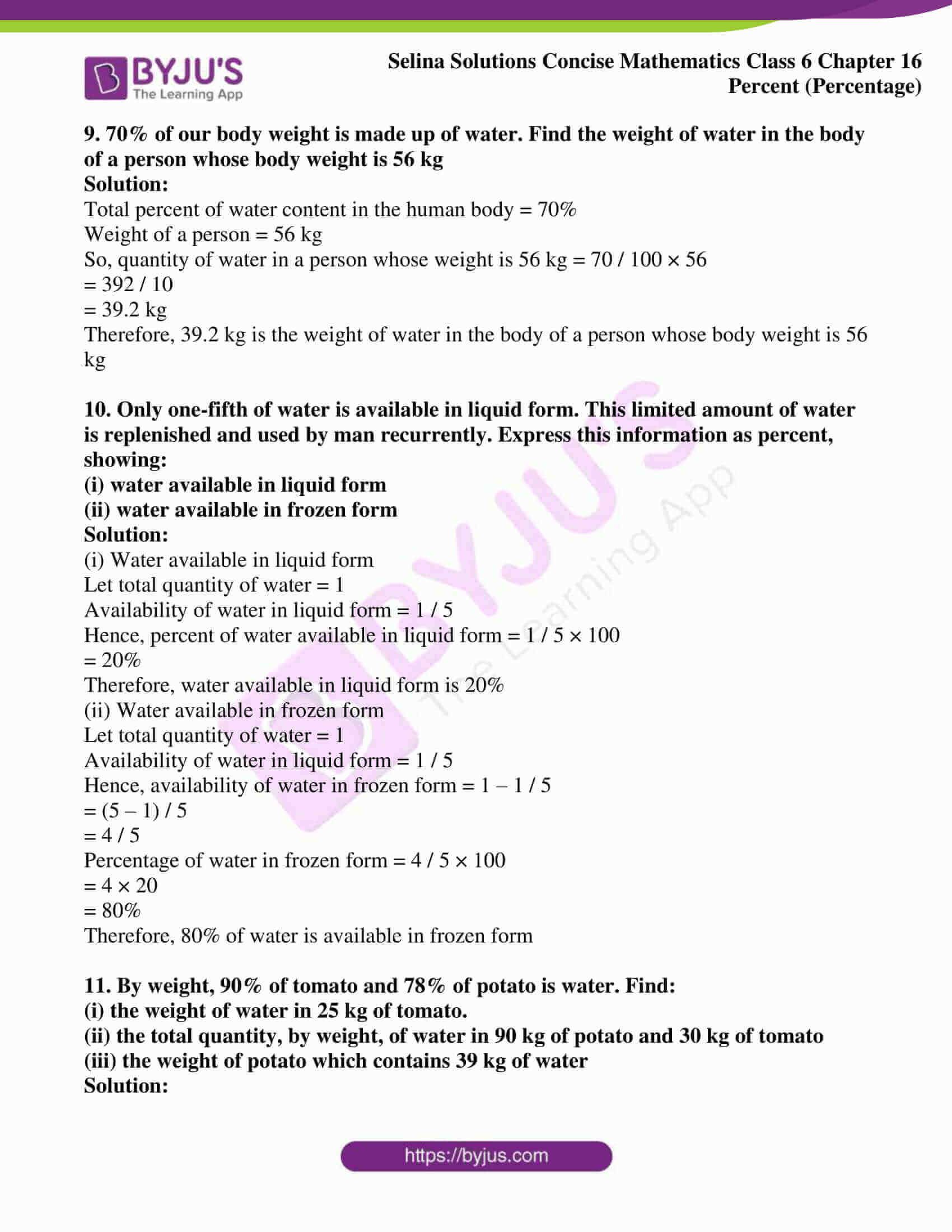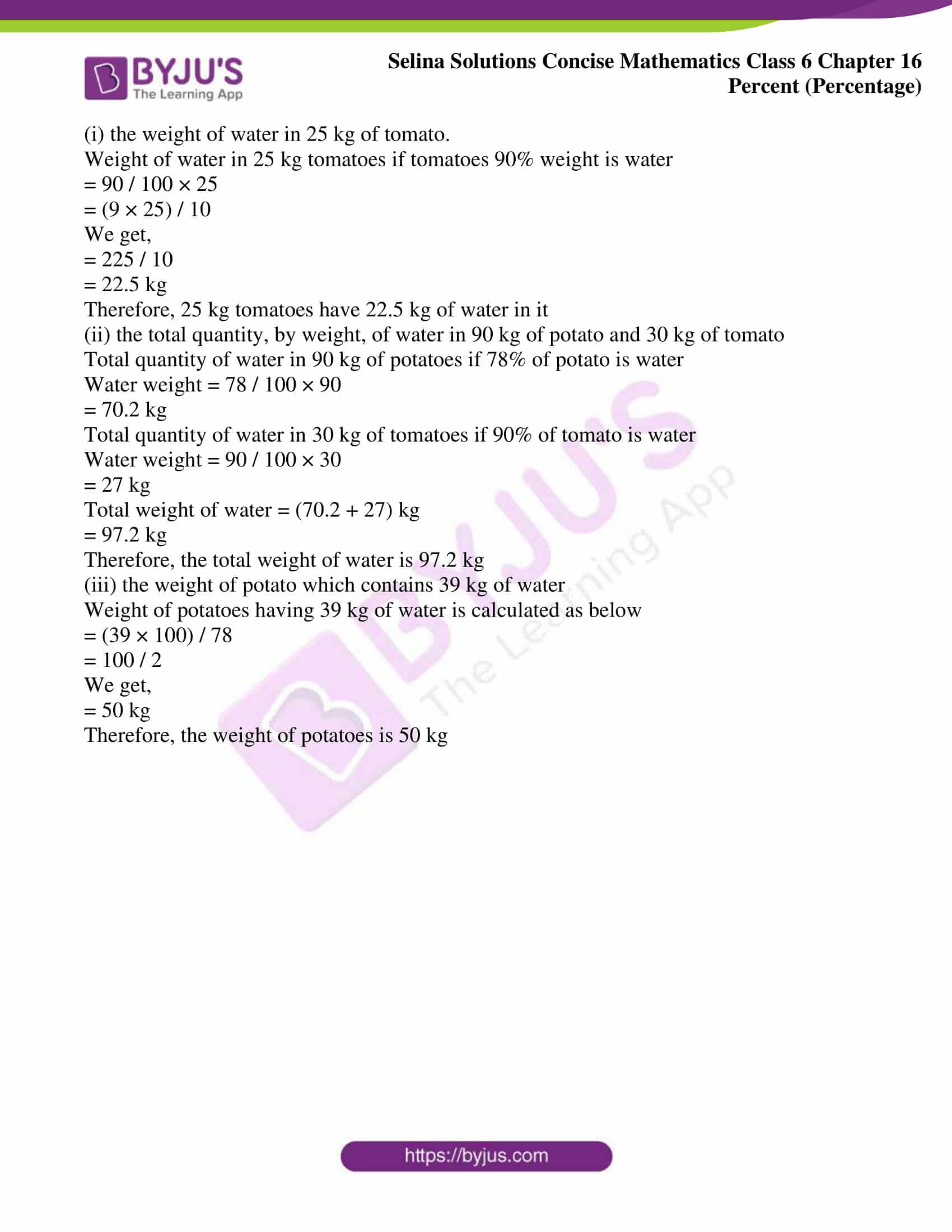### Exercises of Selina Solutions Concise Mathematics Class 6 Chapter 16: Percent (Percentage)

Exercise 16(A) Solutions

Exercise 16(B) Solutions

Exercise 16(C) Solutions

## Access Selina Solutions Concise Mathematics Class 6 Chapter 16: Percent (Percentage)

### Exercise 16(A)

1. Express each of the following statements in the percentage form:

(i) 13 out of 20

(ii) 21 eggs out of 30 are good

Solution:

(i) 13 out of 20

The statement in the percentage form is shown below

13 / 20 × 100 = 13 / 2 × 10

= 13 × 5

= 65%

(ii) The statement in the percentage form is shown below

21 / 30 × 100 = 21 / 3 × 10

= 7 × 10

= 70%

Hence, 70% of eggs are good

2. Express the following fractions as percent:

(i) 3 / 200

(ii) 5 / 6

(iii) 65 / 80

(iv) 2 / 3

Solution:

(i) 3 / 200

Fractions as percentage is shown below

3 / 200 × 100 = 3 / 2

We get,

= 1.5%

(ii) 5 / 6

Fractions as percentage is shown below

5 / 6 × 100 = 500 / 6

On calculating, we get

=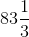%

(iii) 65 / 80

Fractions as percentage is shown below

65 / 80 × 100 = 65 / 8 × 10

= 325 / 4

On further calculation, we get

=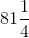%

(iv) 2 / 3

Fractions as percentage is shown below

2 / 3 × 100 = 200 / 3

On calculating, we get

=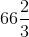%

3. Express as percent:

(i) 0.10

(ii) 0.02

(iii) 0.7

(iv) 0.15

(v) 0.032

Solution:

(i) 0.10

The given decimal expressed in percent is as follows

0.10 × 100 = 10 / 100 × 100

We get,

= 10%

(ii) 0.02

The given decimal expressed in percent is as follows

0.02 × 100 = 2 / 100 × 100

We get,

= 2%

(iii) 0.7

The given decimal expressed in percent is as follows

0.7 × 100 = 7 / 10 × 100

We get,

= 70%

(iv) 0.15

The given decimal expressed in percent is as follows

0.15 × 100 = 15 / 100 × 100

We get,

= 15%

(v) 0.032

The given decimal expressed in percent is as follows

0.032 × 100 = 32 / 1000 × 100

We get,

= 3.2%

4. Convert into fractions in their lowest terms:

(i) 8%

(ii) 20%

(iii) 85%

(iv) 250%

(v) 12 ½ %

Solution:

(i) 8%

Fraction in its lowest form is shown below

8 / 100 = 4 / 50

We get,

= 2 / 25

(ii) 20%

Fraction in its lowest form is shown below

20 / 100 = 2 / 10

We get,

= 1 / 5

(iii) 85%

Fraction in its lowest form is shown below

85 / 100 = 17 / 20

(iv) 250%

Fraction in its lowest form is shown below

250 / 100 = 25 / 10

On further calculation, we get

= 5 / 2

=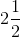(v) 12 ½ %

Fraction in its lowest form is shown below

(25 / 2) % = 25 / 200

On further calculation, we get

= 5 / 40

= 1 / 8

5. Express as decimal fractions:

(i) 25%

(ii) 108%

(iii) 95%

(iv) 4.5%

(v) 29.2%

Solution:

(i) 25%

The statement expressed as decimal fraction is given below

25 / 100

= 0.25

Hence, the decimal fraction of 25% is 0.25

(ii) 108%

The statement expressed as decimal fraction is given below

108 / 100

= 1.08

Hence, the decimal fraction of 108% is 1.08

(iii) 95%

The statement expressed as decimal fraction is given below

95 / 100

= 0.95

Hence, the decimal fraction of 95% is 0.95

(iv) 4.5%

The statement expressed as decimal fraction is given below

4.5 / 100 = 45 / 1000

We get,

= 0.045

Hence, the decimal fraction of 4.5% is 0.045

(v) 29.2%

The statement expressed as decimal fraction is given below

29.2 / 100 = 292 / 1000

We get,

= 0.292

6. Express each of the following natural numbers as percent:

(i) 7

(ii) 2

(iii) 19.5

(iv) 5.37

Solution:

(i) 7

The given natural number expressed in percent is shown below

7 × 100

= 700%

(ii) 2

The given natural number expressed in percent is shown below

2 × 100

= 200%

(iii) 19.5

The given natural number expressed in percent is shown below

19.5 × 100

= 1950%

(iv) 5.37

The given natural number expressed in percent is shown below

5.37 × 100

= 537%

### Exercise 16(B)

1. Express

(i) Rs 5 as a percentage of Rs 25

(ii) 80 paise as a percent of Rs 4

(iii) 700 gm as a percentage of 2.8 kg

(iv) 90 cm as a percent of 4.5 m

Solution:

(i) Rs 5 as a percentage of Rs 25

Rs 5 as a percentage of Rs 25 is given below

5 / 25 × 100

= 5 × 4

= 20%

Hence, Rs 5 is 20% of Rs 25

(ii) 80 paise as a percent of Rs 4

80 paise as a percent of Rs 4 is given below

We know that,

Rs 4 = 400 paisa

80 / 400 × 100

= 80 / 4

= 20%

Hence, 80 paisa is 20% of Rs 4

(iii) 700 gm as a percentage of 2.8 kg

700 gm as a percent of 2.8 kg is given below

We know that,

1 kg = 1000 gm

Thus, 2.8 kg = 2800 gm

So,

700 / 2800 × 100 = 700 / 28

= 100 / 4

We get,

= 25%

Hence, 700 gm is 25% of 2.8 kg

(iv) 90 cm as a percent of 4.5 m

90 cm as a percent of 4.5 m is given below

We know that,

1 m = 100 cm

Thus, 4.5 m = 450 cm

So,

90 / 450 × 100 = 900 / 45

= 20%

Hence, 90 cm is 20% of 4.5 m

2. Express the first quantity as a percent of the second:

(i) 40 P, Rs 2

(ii) 500 gm, 6 kg

(iii) 42 seconds, 6 minutes

Solution:

(i) 40 P, Rs 2

40 P as a percent of Rs 2 is shown below

We know that,

1 Rs = 100 P

So, 2 Rs = 200 P

40 / 200 × 100 = 40 / 2

We get,

= 20%

Hence, 40 P is 20 % of Rs 2

(ii) 500 gm, 6 kg

500 gm as a percent of 6 kg is shown below

We know that,

1 kg = 1000 gm

So, 6 kg = 6000 gm

500 / 6000 × 100 = 500 / 60

= 50 / 6

We get,

= 25 / 3

=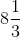%

Hence, 500 gm is% of 6 kg

(iii) 42 seconds 6 minutes

42 seconds as a percent of 6 minutes is shown below

We know that,

1 min = 60 sec

So, 6 min = 360 sec

42 / 360 × 100 = 420 / 36

= 35 / 3

We get,

=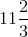%

Hence, 42 sec is% of 6 minutes

3. Find the value of each of the following:

(i) 20% of Rs 150

(ii) 90% of 130

(iii) 15% of 2 minutes

(iv) 7.5% of 500 kg

Solution:

(i) 20% of Rs 150

20% of Rs 150 is given below

20 / 100 × 150 = 2 × 15

We get,

= 30

Hence, 20% of Rs 150 is Rs 30

(ii) 90% of 130

90% of 130 is given below

90 / 100 × 130 = 9 × 13

We get,

= 117

Hence, 90% of 130 is 117

(iii) 15% of 2 minutes

15% of 2 minutes is given below

We know that,

1 min = 60 sec

So, 2 min = 120 sec

15 / 100 × 120 = (15 × 12) / 10

We get,

= 180 / 10

= 18 sec

Hence, 15% of 2 min is 18 sec

(iv) 7.5% of 500 kg

7.5% of 500 kg is given below

7.5 / 100 × 500 = 7.5 × 5

We get,

= 37.5 kg

Hence, 7.5% of 500 kg is 37.5 kg

4. If a man spends 70% of his income, what percent does he save?

Solution:

Let the total income of the man be = Rs 100

If a man spends 70% of his income, then total expense = 70 / 100 × 100

= Rs 70

Savings after his expenditure = 100 – 70 = 30

Hence, his savings in percent = 30 / 100 × 100

= 30%

Therefore, the man saved 30% of his total income

5. A girl gets 65 marks out of 80. What percent marks did she get?

Solution:

Total marks in the exam = 80

Marks obtained by a girl = 65

Her percentage = 65 / 80 × 100

= 650 / 8

We get,

= 81.25%

Therefore, the marks obtained by a girl in percentage is 81.25%

6. A class contains 25 children, of which 6 are girls. What percentage of the class are the boys

Solution:

Total number of children in the class = 25

Number of girls = 6

Number of boys = 25 – 6

= 19 boys

Thus, percentage of boys in the class = 19 / 25 × 100

= 19 × 4

We get,

= 76%

Therefore, there are 76% of boys in the class

7. A tin contains 20 litres of petrol. Due to leakage, 3 litres of petrol is lost. What percent is still present in the tin?

Solution:

Total quantity of petrol in the tin = 20 litres

Quantity of petrol lost, due to leakage = 3 litres

Now, quantity of petrol left in the tin = (20 – 3) = 17 litres

Therefore, percentage of petrol left in the tin = 17 / 20 × 100

= 17 × 5

We get,

= 85%

Thus, 85% of petrol is left in the tin

8. An alloy of copper and zinc contains 45% copper and the rest zinc. Find the weight of zinc in 20 kg of the alloy.

Solution:

Total weight of the alloy = 20 kg

Percentage of copper = 45%

So, percentage of zinc = (100 – 45) %

= 55%

As total quantity is always 100%

Thus, weight of zinc = 55 / 100 × 20 = 110 / 10

= 11 kg

Therefore, the weight of zinc in the alloy is 11 kg

9. A boy got 60 out of 80 in Hindi, 75 out of 100 in English and 65 out of 70 in Arithmetic. In which subject his percentage of marks the best? Also, find his overall percentage

Solution:

Marks obtained by a boy in Hindi out of 80 = 60 marks

So, his percentage in Hindi = 60 / 80 × 100 = 600 / 8

= 75%

Marks obtained by a boy in English out of 100 = 75

So, his percentage in English = 75 / 100 × 100 = 75%

Marks scored by a boy in Arithmetic out of 70 = 65

So, his percentage in Arithmetic = 65 / 70 × 100 = 650 / 7

=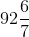%

Clearly, it shows that he gets best marks in Arithmetic

Now,

Total marks of all the three subjects = 80 + 100 + 70

= 250 marks

Total marks obtained by a boy in all the three subjects = 60 + 75 + 65

= 200 marks

His overall percentage = 200 / 250 × 100 = 2000 / 25

= 80%

Hence, the overall percentage of a boy is 80%

10. In a camp, there were 500 soldiers. 60 more soldiers joined them. What percent of the earlier (original) number have joined the camp

Solution:

Total number of soldiers in the camp = 500 soldiers

Number of soldiers joined the camp = 60 soldiers

So, percentage of soldiers joining the camp as per the earlier strength

= 60 / 500 × 100

= 60 / 5

We get,

= 12%

Therefore, 12% of soldiers joined the camp as per the earlier strength

11. In a plot of ground of area 6000 sq. m, only 4500 sq. m is allowed for construction. What percent is to be left without construction?

Solution:

Total area of the plot = 6000 sq. m

Area of plot allowed for construction = 4500 sq. m

So, area of plot left = (6000 – 4500) sq. m

= 1500

Thus, area of plot left in percentage = 1500 / 6000 × 100

= 150 / 6

We get,

= 25%

Therefore, 25% of plot is left without construction

12. Mr. Sharma has a monthly salary of Rs 8000. If he spends Rs 6400 every month, find:

(i) his monthly expenditure as percent

(ii) his monthly savings as percent

Solution:

(i) his monthly expenditure as percent

Total salary of Mr. Sharma in a month = Rs 8000

Money spent by him in every month = Rs 6400

So, his expenditure percentage = 6400 / 8000 × 100 = 640 / 8

= 80%

Therefore, Mr. Sharma spends 80% of his salary

(ii) his monthly savings as percent

Total salary of Mr. Sharma in a month = Rs 8000

Money spent by him in every month = Rs 6400

So, His monthly saving = Rs (8000 – 6400)

= Rs 1600

His savings in percentage = 1600 / 8000 × 100

= 160 / 8

= 20%

Therefore, Mr. Sharma saves 20% of his salary

13. The monthly salary of Rohit is Rs 24000. If his salary increases by 12%, find his new monthly salary

Solution:

Monthly salary of Rohit = Rs 24000

Percent increased in his salary = 12%

Thus, increase in his salary = 12 / 100 × 24000

= 12 × 240

= Rs 2880

Hence, his new salary is = 24000 + 2880

= Rs 26880

Therefore, the new salary of Rohit is Rs 26880

14. In a sale, the price of an article is reduced by 30%. If the original price of the article is Rs 1800, find:

(i) the reduction in the price of the article

(ii) reduced price of the article

Solution:

(i) the reduction in the price of the article

Original price of an article = Rs 1800

The price of an article reduced by = 30%

Hence, reduction in the price = 30 / 100 × 1800

= 30 × 18

= 540

Therefore, the price of an article reduced by Rs 540

(ii) reduced price of the article

Original price of an article = Rs 1800

Reduction in price = Rs 540

Hence, the final price = Rs (1800 – 540)

= Rs 1260

Therefore, the final price of the article is Rs 1260

15. Evaluate:

(i) 30% of 200 + 20% of 450 – 25% of 600

(ii) 10% of Rs 450 – 12% of Rs 500 + 8% of Rs 500

Solution:

(i) 30% of 200 + 20% of 450 – 25% of 600

= (30 / 100 × 200) + (20 / 100 × 450) – (25 / 100 × 600)

= (30 × 2) + (2 × 45) – (25 × 6)

We get,

= 60 + 90 – 150

= 150 – 150

= 0

(ii) 10% of Rs 450 – 12% of Rs 500 + 8% of Rs 500

= (10 / 100 × 450) – (12 / 100 × 500) + (8 / 100 × 500)

= (1 × 45) – (12 × 5) + (8 × 5)

We get,

= 45 – 60 + 40

= 85 – 60

= Rs 25

### Exercise 16(C)

1. The price of rice rises from Rs 30 per kg to Rs 36 per kg. Find the percentage rise in the price of rice

Solution:

Initial price of rice = Rs 30

Price increased in rice = Rs 36

So, the percent increase in price

= 6 / 30 × 100

= (6 × 10) / 3

We get,

= 20%

Therefore, the price of rice increased by 20%

2. The population of a small locality was 4000 in 1979 and 4500 in 1981, By what percent had the population increase?

Solution:

Population of a small locality in 1979 = 4000

Population of a small locality in 1981 = 4500

So, increase in population = 4500 – 4000

= 500

Thus, percent increase in population

= 500 / 4000 × 100

= 50 / 4

We get,

= 12.5%

Therefore, the population of the locality increased by 12.5% in two years

3. The price of a scooter was Rs 8000 in 1975. It came down to Rs 6000 in 1980. By what percent had the price of the scooter came down?

Solution:

Price of a scooter in 1975 = Rs 8000

Price of a scooter in 1980 = Rs 6000

So, reduction in the price = Rs 8000 – Rs 6000

= Rs 2000

Hence, percent decrease in price

= 2000 / 8000 × 100

= 200 / 8

We get,

= 25%

Therefore, the price of a scooter reduced by 25%

4. Find the resulting quantity when:

(i) Rs 400 is decreased by 8%

(ii) 25 km is increased by 5%

(iii) a speed of 600 km/h is increased by 12 ½ %

(iv) there is 2.5% increase in a salary of Rs 62, 500

Solution:

(i) Rs 400 is decreased by 8%

Rs 400 decreased by 8% is shown below

8 / 100 × 400

= 8 × 4

= Rs 32

Hence, decreased amount = Rs (400 – 32)

= Rs 368

(ii) 25 km is increased by 5%

5% increased to 25 km is shown below

5% of 25 km = 5 / 100 × 25

= 1.25 km

Increased distance = 25 km + 1.25 km

= 26.25 km

Therefore, increase in distance is 26.25 km

(iii) a speed of 600 km/h is increased by 12 ½ %

12 ½ % Increased to 600 km/h is as shown below

12 ½ % = 25 / 2 of 600 km/h

= 25 / 200 × 600

= 25 × 3

We get,

= 75 km/h

Hence, the increase in speed is calculated as below

= 600 km/h + 75 km/h

= 675 km/h

Therefore, the increased speed is 675 km/h

(iv) there is 2.5% increase in a salary of Rs 62, 500

2.5% increased to Rs 62,500 salary is calculated as below

2.5% of Rs 62500

= [25 / (10 × 100)] × 62500

We get,

= (25 × 625) / 10

On further calculation, we get

= 15625 / 10

= Rs 1562.50

Hence, final salary

= Rs 62500 + Rs 1562.50

= Rs 64062.50

Therefore, the final salary is Rs 64062.50

5. The population of a village decreased by 12%. If the original population was 25000, find the population after decrease?

Solution:

The original population of the village = 25000

Percent decrease in population = 12%

12% of 25000

= 12/100 × 25000

= 12 × 250

We get,

= 3000

Final population = 25000 – 3000

= 22000

Therefore, the final population of the village is 22000

6. Out of a salary of Rs 13,500, I keep 1 / 3 as savings. Of the remaining money, I spend 50% on food and 20% on house rent. How much do I spend on food and house rent?

Solution:

My total salary = Rs 13500

One-third of the salary for saving = 1 / 3 × Rs 13500

= Rs 4500

Remaining money = Rs 13500 – Rs 4500

= Rs 9000

Amount spend on food = 50%

= 50 / 100 × 9000

= 50 × 90

= 4500

Amount spend on house rent = 20%

= 20 / 100 × 9000

= 20 × 90

= Rs 1800

Hence, total amount spend on both food and house rent

= Rs 4500 + Rs 1800

= Rs 6300

Therefore, total amount spend on food and house rent is Rs 6300

7. A tank can hold 50 litres of water. At present, it is only 30% full. How many litres of water shall I put into the tank so that it becomes 50% full?

Solution:

Total quantity of water a tank can hold = 50 litres

Water quantity at present = 30%

= 30 / 100 × 50

= 3 × 5

= 15 litres

Hence, 30% of the total capacity = 15 litres

50% of the total capacity = 50 / 100 × 50

= 5 × 5

= 25 litres

Hence, water required filling the tank to 50% = (25 – 15) litre

= 10 litres

Therefore, 10 litres of water is required to fill the tank to 50% full

8. In an election, there are a total of 80000 voters and two candidates, A and B. 80% of the voters go to the polls out of which 60% vote for A. How many votes does B get.

Solution:

Total number of voters = 80000

Total number of votes polled = 80%

= 80 / 100 × 80000

= 80 × 800

= 64000

= 100% – 60%

= 40%

Hence, total number of votes received by B = 40 / 100 × 64000

= 40 × 640

We get,

= 25600

9. 70% of our body weight is made up of water. Find the weight of water in the body of a person whose body weight is 56 kg

Solution:

Total percent of water content in the human body = 70%

Weight of a person = 56 kg

So, quantity of water in a person whose weight is 56 kg = 70 / 100 × 56

= 392 / 10

= 39.2 kg

Therefore, 39.2 kg is the weight of water in the body of a person whose body weight is 56 kg

10. Only one-fifth of water is available in liquid form. This limited amount of water is replenished and used by man recurrently. Express this information as percent, showing:

(i) water available in liquid form

(ii) water available in frozen form

Solution:

(i) Water available in liquid form

Let total quantity of water = 1

Availability of water in liquid form = 1 / 5

Hence, percent of water available in liquid form = 1 / 5 × 100

= 20%

Therefore, water available in liquid form is 20%

(ii) Water available in frozen form

Let total quantity of water = 1

Availability of water in liquid form = 1 / 5

Hence, availability of water in frozen form = 1 – 1 / 5

= (5 – 1) / 5

= 4 / 5

Percentage of water in frozen form = 4 / 5 × 100

= 4 × 20

= 80%

Therefore, 80% of water is available in frozen form

11. By weight, 90% of tomato and 78% of potato is water. Find:

(i) the weight of water in 25 kg of tomato.

(ii) the total quantity, by weight, of water in 90 kg of potato and 30 kg of tomato

(iii) the weight of potato which contains 39 kg of water

Solution:

(i) the weight of water in 25 kg of tomato.

Weight of water in 25 kg tomatoes if tomatoes 90% weight is water

= 90 / 100 × 25

= (9 × 25) / 10

We get,

= 225 / 10

= 22.5 kg

Therefore, 25 kg tomatoes have 22.5 kg of water in it

(ii) the total quantity, by weight, of water in 90 kg of potato and 30 kg of tomato

Total quantity of water in 90 kg of potatoes if 78% of potato is water

Water weight = 78 / 100 × 90

= 70.2 kg

Total quantity of water in 30 kg of tomatoes if 90% of tomato is water

Water weight = 90 / 100 × 30

= 27 kg

Total weight of water = (70.2 + 27) kg

= 97.2 kg

Therefore, the total weight of water is 97.2 kg

(iii) the weight of potato which contains 39 kg of water

Weight of potatoes having 39 kg of water is calculated as below

= (39 × 100) / 78

= 100 / 2

We get,

= 50 kg

Therefore, the weight of potatoes is 50 kg